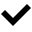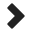# 8. Mongoose (Terminology of Mongoose)

Objectives :In the this section, we’re going to look at some of the basic terminologies in mongoose.

• Schema
• Model

# Terminology of Mongoose

Mongoose will use the terms Schema and Model. While doing any operation, in this entire tutorial we will use the terms ‘Schema’ and ‘Model’ frequently so we need to know. lets know those

## What is Schema?“Mongoose Schema will create a mongodb collection and defines the shape of the documents within that collection”.If we want to create a mongodb collection in a structured manner similar to SQL tables then we can do that using mongoose Schema.In the schema creation we will specify the details of fields like field name, its type, default value if need, index concept if need, constraint concept if need.
Like

 `1` `var` `userSchema = ``new` `Schema({`
 `2` `userid : String,`
 `3` `chips : {type:double, ``default``:0.0},`
 `4` `regdate : {type:Date, ``default``: Date.now,required:``true``}`
 `5` `})`

Simply Schema creation is similar to table creation of SQL.

Note:-

• A Schema can contains static methods
• A Schema can contains non-static(instance) methods
• A Schema can contains indexes

## Model:-Schemas define a structure we will apply that Schema part to a model, means “Models are the constructors compiled from our schema definitions”.Instances of these models represent documents which can be saved and retrieved from our database. All document creation and retrieval from the database is handled by these models.We can create the Models using mongoose.model() constructor as

var modelinstance= mongoose.model(‘collection-name’,schemaname);

for the above ‘userSchema’ example check the model creation

var userModel = mongoose.model(‘users’,userSchema);How to create document instances using model’s

var docinstance = new modelinstance(values for schema );

Example:-

 `1` `var` `alex = ``new` `userModel({userid:``'Alex'``,chips:10000,regdate:Date.now});`
 `2` `var` `michal = ``new` `userModel({userid:``'Michal'` `});`